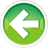8.3 — Numeric conversions

In the previous lesson (8.2 -- Floating-point and integral promotion), we covered numeric promotions, which are conversions of specific narrower numeric types to wider numeric types (typically int or double) that can be processed efficiently.

C++ supports another category of numeric type conversions, called numeric conversions, that cover additional type conversions not covered by the numeric promotion rules.

Key insight

Any type conversion covered by the numeric promotion rules (8.2 -- Floating-point and integral promotion) is a numeric promotion, not a numeric conversion.

There are five basic types of numeric conversions.

1) Converting an integral type to any other integral type (excluding integral promotions):

2) Converting a floating point type to any other floating point type (excluding floating point promotions):

3) Converting a floating point type to any integral type:

4) Converting an integral type to any floating point type:

5) Converting an integral type or a floating point type to a bool:

As an aside...

Because brace initialization disallows some numeric conversions (more on this is a moment), we use copy initialization in this lesson (which does not have any such limitations) in order to keep the examples simple.

Narrowing conversions

Unlike a numeric promotion (which is always safe), a numeric conversion may (or may not) result in the loss of data or precision.

Some numeric conversions are always safe (such as int to long, or int to double). Other numeric conversions, such as double to int, may result in the loss of data (depending on the specific value being converted and/or the range of the underlying types):

In C++, a narrowing conversion is a numeric conversion that may result in the loss of data. Such narrowing conversions include:

• From a floating point type to an integral type.
• From a wider floating point type to a narrower floating point type, unless the value being converted is constexpr and is in range of the destination type (even if it can not be represented exactly).
• From an integer to a floating point type, unless the value being converted is constexpr and is in range of the destination type and can be converted back into the original type without data loss.
• From a wider integer type to a narrower integer type, unless the value being converted is constexpr and after integral promotion will fit into the destination type.

The good news is that you don’t need to remember these. Your compiler will usually issue a warning (or error) when it determines that an implicit narrowing conversion is required.

Warning

Compilers will often not warn when converting a signed int to an unsigned int, or vice-versa, even though these are narrowing conversions. Be extra careful of inadvertent conversions between these types (particularly when passing an argument to a function taking a parameter of the opposite sign).

For example, when compiling the following program:

Visual Studio produces the following warning:

warning C4244: 'initializing': conversion from 'double' to 'int', possible loss of data

In general, narrowing conversions should be avoided, but there are situational cases where you might need to do one. In such cases, you should make the implicit narrowing conversion explicit by using static_cast. For example:

Best practice

Avoid narrowing conversions whenever possible. If you do need to perform one, use static_cast to make it an explicit conversion.

Brace initialization disallows narrowing conversions

Narrowing conversions are strictly disallowed when using brace initialization (which is one of the primary reasons this initialization form is preferred):

Visual Studio produces the following error:

error C2397: conversion from 'double' to 'int' requires a narrowing conversion

More on numeric conversions

The specific rules for numeric conversions are complicated and numerous, so here are the most important things to remember.

In all cases, converting a value into a type whose range doesn’t support that value will lead to results that are probably unexpected. For example:

In this example, we’ve assigned a large integer to a variable with type char (that has range -128 to 127). This causes the char to overflow, and produces an unexpected result:

48

Converting from a larger integral or floating point type to a smaller type from the same family will generally work so long as the value fits in the range of the smaller type. For example:

This produces the expected result:

2
0.1234

In the case of floating point values, some rounding may occur due to a loss of precision in the smaller type. For example:

In this case, we see a loss of precision because the float can’t hold as much precision as a double:

0.123456791

Converting from an integer to a floating point number generally works as long as the value fits within the range of the floating point type. For example:

This produces the expected result:

10

Converting from a floating point to an integer works as long as the value fits within the range of the integer, but any fractional values are lost. For example:

In this example, the fractional value (.5) is lost, leaving the following result:

3

While the numeric conversion rules might seen scary, in reality the compiler will generally warn you if you try to do something dangerous (excluding some signed/unsigned conversions).8.4 -- Arithmetic conversionsIndex8.2 -- Floating-point and integral promotion